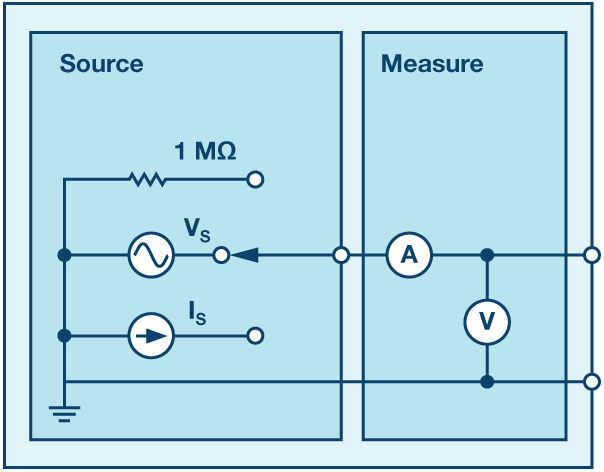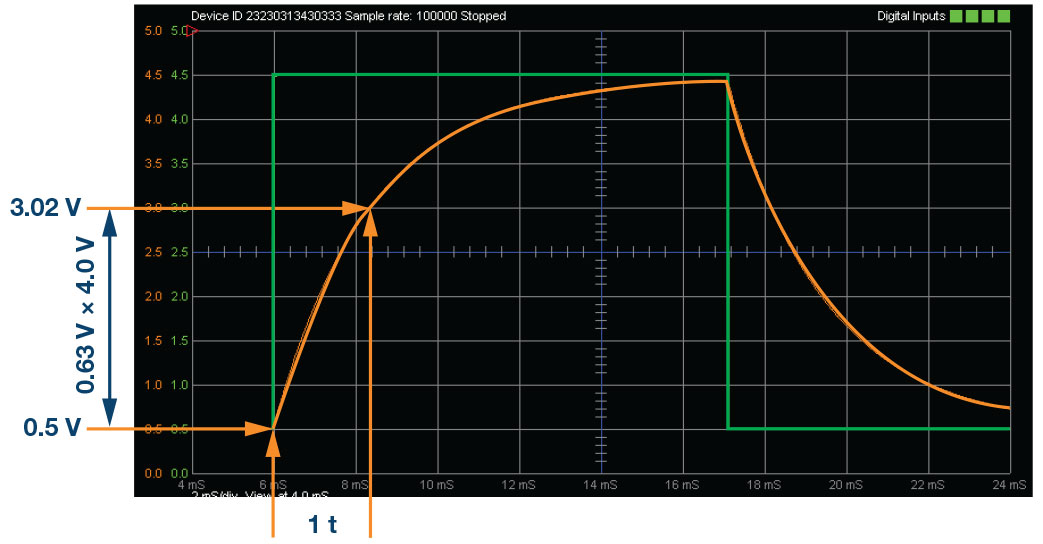# ADALM1000 SMU Training Topic 4: Transient Response of RC Circuit

After the introduction of the SMU ADALM1000 in our Analog Dialogue December 2017 article, we want to continue with the fourth part of our series with some small, basic measurements. You can find the first ADALM1000 article here.Figure 1. A schematic of the ADALM1000.

Now let’s get started with the next experiment.

### Objective:

The objective of this lab activity is to study the transient response of a series RC circuit and understand the time constant concept using pulse waveforms.

### Background:

In this lab activity, you will apply a pulse waveform to the RC circuit to analyze the transient response of the circuit. The pulse width relative to a circuit’s time constant determines how it is affected by an RC circuit.

Time Constant (τ): A measure of time required for certain changes in voltages and currents in RC and RL circuits. Generally, after four time constants (4 τ), the capacitor in the RC circuit is virtually fully charged and the voltage across the capacitor is now approximatively at 98% of its maximum value. This interval is considered to be the transient response of the circuit. When the elapsed time exceeds five time constants (5 τ) after switching has occurred, the currents and voltages have reached their final value, which is also called steady-state response.

Table 1 shows the voltage and current percentage values for the capacitor in the RC charging circuit at a given time constant while charging.

 Time Constant (τ) Percentage of Maximum Voltage Current 0.5 τ 39.3% 60.7% 0.7 τ 50.3% 49.7% τ 63.2% 36.8% 2 τ 86.5% 13.5% 3 τ 95.0% 5.0% 4 τ 98.2% 1.8% 5 τ 99.3% 0.7%

Note that the capacitor will never become 100% charged in reality. Therefore, five time constants are used to consider a capacitor fully charged for all practical purposes.

The time constant of an RC circuit is the product of equivalent capacitance and the Thévenin resistance as viewed from the terminals of the equivalent capacitor.

A pulse is a voltage or current that changes from one level to another and back again. If a waveform’s high time equals its low time it is called a square wave. The length of each cycle of a pulse is its period (T).

The pulse width (tp) of an ideal square wave is equal to half the time period.

The relation between pulse width and frequency is then given by,Figure 2. Series RC circuit.

From Kirchhoff’s laws, it can be shown that the charging voltage VC (t) across the capacitor is given by:

where V is the applied source voltage to the circuit for τ = 0, and RC = τ is the time constant.

The response curve increases and is shown in Figure 3.Figure 3. Capacitor charging for series RC circuit to a step input with time axis normalized by τ.

The discharge voltage for the capacitor is given by:

Where Vo is the initial voltage stored in capacitor at t = 0, and RC = τ is time constant. The response curve is a decaying exponential as shown in Figure 4.Figure 4. Capacitor discharging for series RC circuit.

### Materials:

• ADALM1000 hardware module
• Resistors (2.2 kΩ, 10 kΩ)
• Capacitors (1 μF, 0.01 μF)

### Procedure:

1. Set up the circuit shown in Figure 5 on your solderless breadboard with the component values R1 = 2.2 kΩ and C1 = 1 μF. Open the ALICE Oscilloscope software.
2. Set the Channel A arbitrary waveform generator (AWG) Min value to 0.5 V and the Max value to 4.5 V to apply a 4 V p-p square wave centered on 2.5 V as the input voltage to the circuit. From the AWG A Mode drop down menu, select the SVMI mode. From the AWG A Shape drop down menus select Square. From the AWG B Mode drop down menu, select the Hi-Z mode.Figure 5. Breadboard diagram of an RC circuit.Figure 6. Breadboard connections for RC circuit R1 = 2.2 kΩ and C1 = 1 μF.
3. From the ALICE Curves drop down menu, select CA-V and CB-V for display. From the Trigger drop down menu, select CA-V and Auto Level. Adjust the time base until you have at approximately two cycles of the square wave on the display grid.Figure 7. Oscilloscope configuration. This configuration uses the oscilloscope to look at the input of the circuit on Channel A and the output of the circuit on Channel B. Make sure you have checked the Sync AWG selector.
4. Observe the response of the circuit for the following three cases and record the results.

1. Pulse width » 5 τ: Set the frequency of AWG A output such that the capacitor has enough time to fully charge and discharge during each cycle of the square wave. Let the pulse width be 15 τ and set the frequency according to Equation 2. The value you have found should be approximately 15 Hz. Determine the time constant from the waveforms obtained on the screen if you can. If you cannot obtain the time constant easily, explain possible reasons.
2. Pulse width = 5 τ: Set the frequency such that the pulse width = 5 τ (this should be approximately 45 Hz). Since the pulse width is 5 τ, the capacitor should just be able to fully charge and discharge during each pulse cycle (see Figure 3 and Figure 4).Figure 8. Measuring the time constant t approximately by counting the number of squares.
3. Pulse width « 5 τ: In this case the capacitor does not have time to charge significantly before it is switched to discharge, and vice versa. Let the pulse width be only 1.0 τ in this case and set the frequency accordingly.
5. Repeat the procedure using R1 = 10 kΩ and C1 = 0.01 μF and record the measurements.

### Questions:

1. Calculate the time constant (τ) using Equation 1 and compare it to the measured value from 4b (R = 2.2 kΩ and C = 0.01 μF).
2. Repeat this for another set of values, R = 10 kΩ and C = 0.01 μF.

You can find the answers at the StudentZone blog.

### Notes

As in all the ALM labs, we use the following terminology when referring to the connections to the ALM1000 connector and configuring the hardware. The green shaded rectangles indicate connections to the ADALM1000 analog I/O connector. The analog I/O channel pins are referred to as CA and CB. When configured to force voltage/measure current, –V is added (as in CA-V) or when configured to force current/measure voltage, –I is added (as in CA-I). When a channel is configured in the high impedance mode to only measure voltage, –H is added (as in CA-H).

Scope traces are similarly referred to by channel and voltage/current, such as CA-V and CB-V for the voltage waveforms, and CA-I and CB-I for the current waveforms.

We are using the ALICE Rev 1.1 software for those examples here.

File: alice-desktop-1.1-setup.zip. Please download here.

The ALICE desktop software provides the following functions:

• A 2-channel oscilloscope for time domain display and analysis of voltage and current waveforms.
• The 2-channel arbitrary waveform generator (AWG) controls.
• The X and Y display for plotting captured voltage and current vs. voltage and current data, as well as voltage waveform histograms.
• The 2-channel spectrum analyzer for frequency domain display and analysis of voltage waveforms.
• The Bode plotter and network analyzer with built-in sweep generator.
• An impedance analyzer for analyzing complex RLC networks and as an RLC meter and vector voltmeter.
• A dc ohmmeter measures unknown resistance with respect to known external resistor or known internal 50 Ω.
• Board self-calibration using the AD584 precision 2.5 V reference from the ADALP2000 analog parts kit.
• ALICE M1K voltmeter.
• ALICE M1K meter source.
• ALICE M1K desktop tool.

For more information, please look here.

Note: You need to have the ADALM1000 connected to your PC to use the software.Figure 9. ALICE desktop 1.1 menu.